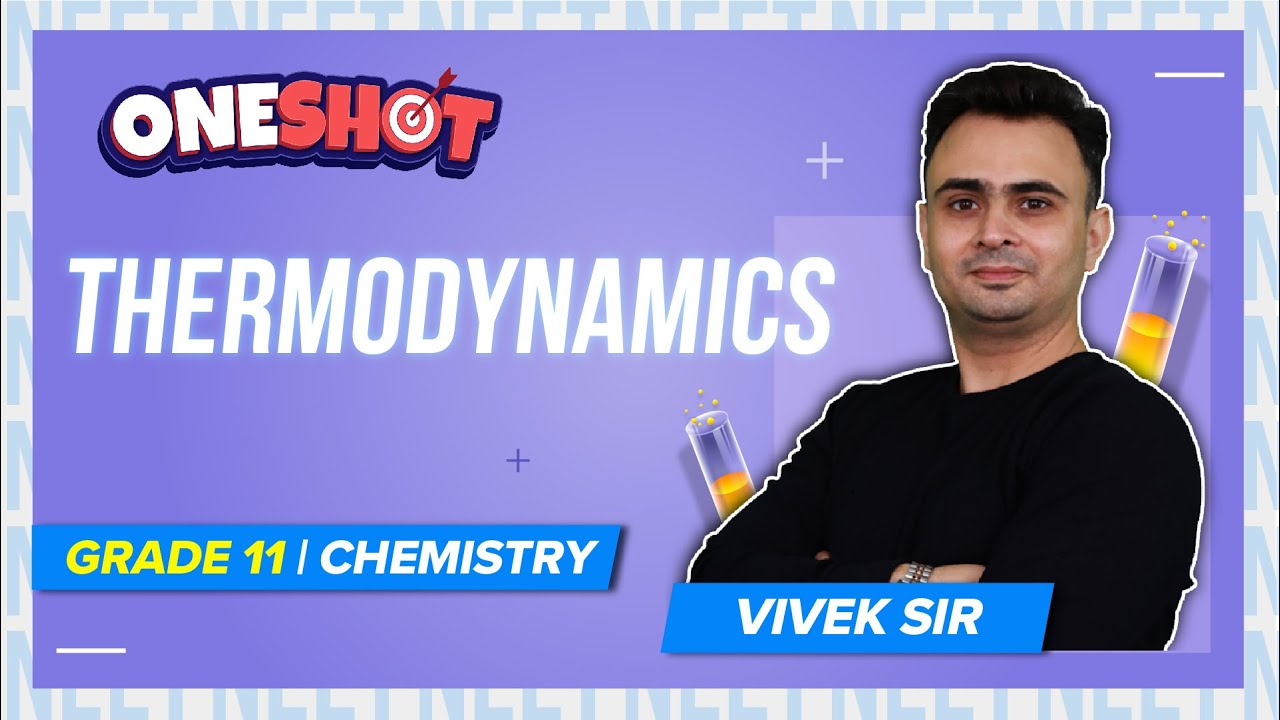# MCQs on Thermodynamics for NEET

Thermodynamics is the study of energy transformations. The chemical energy present in a molecule is released in various reactions. The chemical energy can be transformed into other forms of energy, e.g. to do mechanical work as burning of fuel in an engine, provide electrical energy as in dry cell, etc. Thermodynamics takes into account the initial and final state of a system and does not consider the rate and method by which changes occur. The laws of thermodynamics deal with a macroscopic system present in equilibrium or changing from one state of equilibrium to another.

1. What will be the value of ΔH, if the forward and reverse reactions have the same energy of activation?

(a) ΔH = ΔG = ΔS = 0

(b) ΔS = 0

(c) ΔG = 0

(d) ΔH = 0

2. What will be the entropy change (ΔS), when an ideal gas undergoes a change in the pressure from pi to pf isothermally?

(a)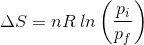(b)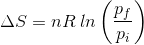(c)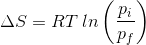(d)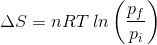3. Find out the relationship between x, y and z considering below three reactions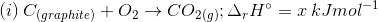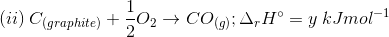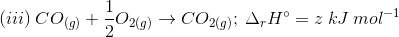(a) y = 2z – x

(b) x = y – z

(c) x = y + z

(d) z = x + y

4. What is the molar entropy change for melting of ice at 0℃, if ΔHf = 1.435 kcal/mol?

(a) 0.526 cal/(mol K)

(b) 5.26 cal/(mol K)

(c) 10.52 cal/(mol K)

(d) 21.04 cal/(mol K)

5. What is the function of a catalyst in a chemical reaction?

(a) decrease rate constant of reaction

(b) increases activation energy of reaction

(c) reduces enthalpy of reaction

(d) does not affect the equilibrium constant of reaction

6. What will be the work done by 3 moles of an ideal gas when it expands spontaneously in a vacuum?

(a) zero

(b) infinite

(c) 3 joules

(d) 9 joules

7. Find the temperature at which the below reaction will be in equilibrium if the enthalpy and entropy change for the reaction is 30 kJ mol-1 and 105 J K-1 mol-1 respectively

Br2(l) + Cl2(g) → 2BrCl(g)

(a) 273 K

(b) 300 K

(c) 450 K

(d) 285.7 K

8. Which is true for the entropy of a spontaneous reaction?

(a) ΔS(system) – ΔS(surroundings) > 0

(b) ΔS(system) + ΔS(surroundings) > 0

(c) ΔS(surroundings) > 0 only

(d) ΔS(system) > 0 only

9. An ideal gas is expanded isothermally at 300 K from 1 litre to 10 litres. Find the ΔE for this process (R = 2 cal mol-1 K-1)

(a) 9 L atm

(b) 1381.1 cal

(c) zero

(d) 163.7 cal

10. ΔH for the reaction N2 + 3H2 ⇋ 2NH3

(a) ΔE – 2RT

(b) ΔE + 2RT

(c) ΔE – RT

(d) ΔH = RT